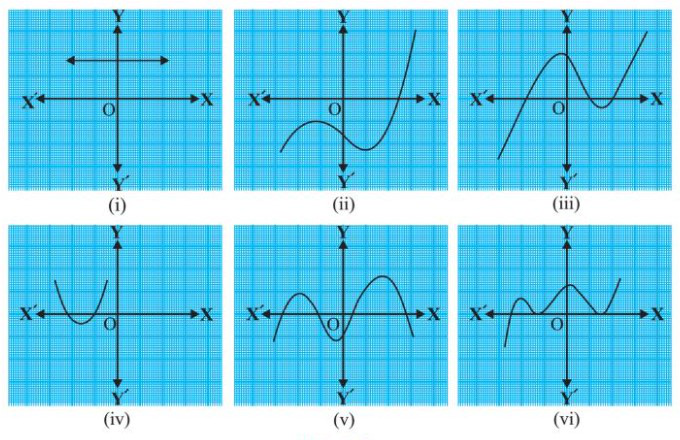# Class 10 NCERT Solutions- Chapter 2 Polynomials – Exercise 2.1

• Last Updated : 28 Dec, 2020

### Question 1. The graphs of y = p(x) are given in Fig. 2.10 below, for some polynomials p(x). Find the number of zeroes of p(x), in each case.Solution:

Attention reader! All those who say programming isn't for kids, just haven't met the right mentors yet. Join the  Demo Class for First Step to Coding Coursespecifically designed for students of class 8 to 12.

The students will get to learn more about the world of programming in these free classes which will definitely help them in making a wise career choice in the future.

So, we have equation y = p(x). When p(x) = 0, y = 0.

That means we only need to find the number of times we get y = 0 in the graph.

In other words, the number of times the graph intersects the x-axis is the answer.

(i) 0. Because the graph doesn’t intersect the x-axis.

(ii) 1. Because the graph intersects the x-axis only at one point.

(iii) 3. Because the graph intersects the x-axis at three points.

(iv) 2. Because the graph intersects the x-axis at two points.

(v) 4. Because the graph intersects the x-axis at four points.

(vi) 3. Because the graph intersects the x-axis at three points.

My Personal Notes arrow_drop_up